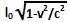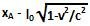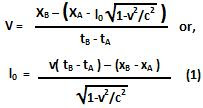## Tuesday, November 17, 2009

### Irodov Problem 1.352

a) Let the proper length of the rod be lo. Since the rod is moving at a speed v with respect to frame K, the length of the rod as seen from Frame K would be lesser than its proper length and equal to. At time instant tA, the front end of the rod was at xA. This implies that the rear end must have been at. At time tB, the rear end of the rod was at xB, this implies that the rear end of the rod moved a distance ofin a time interval of tB - tA. Since the rod was moving at a speed v, we have,b) In order to determine the time interval required to measure the proper length of the rod in equation (1) we can set xB - xA = lo, and we have,